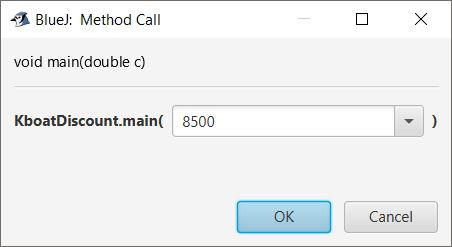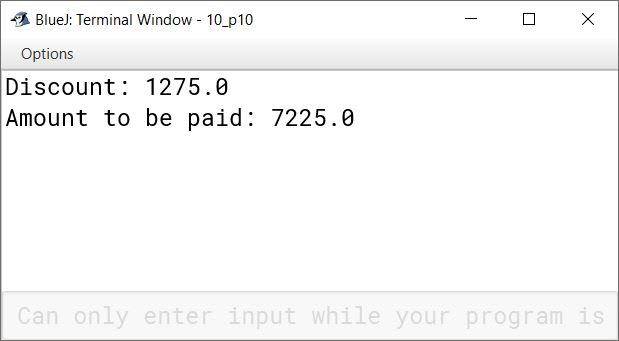# Compiling and Executing Programs

## Choose the correct option

#### Question 1

Which of the following is a valid comment in Java language?

1. /*comment*//
2. /* comment
3. //comment
4. */ comment */

//comment

Reason — In Java, the comment statements are written using double slash (//).

#### Question 2

Which of the following is used to enclose a set of statements to form a compound statement in Java program?

1. Parenthesis
2. Brackets
3. Curly brackets
4. All of them

Curly brackets

Reason — A set of two or more statements placed within curly brackets and executed together is called compound statement.

Note — Here, there is a misprint in part (3). Curly bracket has been misprinted as square brackets.

#### Question 3

Which of the following operators is essentially required while writing if statement?

1. Arithmetic
2. Relational
3. Bitwise
4. All of them

Relational

Reason — In if statement, to test whether a condition is true or not, Relational operators are used.

#### Question 4

Due to which of the following errors, a program does not provide correct result although it has no grammatical mistake?

1. Logical
2. Syntax
3. Type mismatch
4. None of the above

Logical

Reason — Logical error occurs when the logic is incorrect. Thus, the program does not produce the desired result although it is syntactically correct and compiles and executes fine.

#### Question 5

A Java statement is given as:
if((a!=b)&&(a==c))

Which of the following statements is true?

1. b is the smallest number
2. b is the greatest number
3. a is the smallest number
4. Both (a) and (b)

Both (a) and (b)

Reason — The if condition checks that a and b are unequal and a and c are equal. Both these conditions should be true then only if statement will execute. If a and b are unequal and a and c are equal then b will either be the smallest number or the greatest number.

#### Question 6

Which of the following logical operators results in true when all the connecting conditions are true?

1. !
2. ||
3. &&
4. !=

&&

Reason — Logical AND results in true when all the connecting conditions are true.

## State True or False

#### Question 1

Java programs can be compiled and executed on BlueJ platform.
True

#### Question 2

In assignment, the data values are readily available in Java program.
True

#### Question 3

The control skips some lines in a normal flow of control.
False

#### Question 4

In a decision making program, 'if' checks whether the condition is true or false.
True

#### Question 5

You can't assign data values in the main( ) function.
False

#### Question 6

In an if-else statement, the condition is to be defined only with if and not with else.
True

## Predict the output of the given snippets, when executed

#### Question 1

```int x = 1,y = 1;
if(n>0)
{
x=x+1;
y=y+1;
}           ```

What will be the value of x and y, if n assumes a value (i) 1 (ii) 0?

##### Output

(i) When n is 1:
x is 2 and y is 2

(ii) When n is 0:
x is 1 and y is 1

##### Explanation

When n is 1, `if (n>0)` is true. So the statements `x=x+1;` and `y=y+1;` are executed making the values of x and y as 2.

When n is 0, `if (n>0)` is false. Statements `x=x+1;` and `y=y+1;` are not executed so values of x and y remain 1.

#### Question 2

```int b=3,k,r;
float a=15.15,c=0;
if(k==1)
{
r=(int)a/b;
System.out.println(r);
}
else
{
c=a/b;
System.out.println(c);
}```

Syntax Error

##### Explanation

There are 2 syntax errors in this program:

1. Variable k is not initialized with any value before using it in the if check.
2. The statement `float a=15.15` has a syntax error. We are trying to assign a double literal to a float variable. The correct statement will be `float a=15.15f`.

## Correct the errors in the given programs

#### Question 1

```class public
{
public static void main(String args{})
{
int a=45,b=70,c=65.45;
sum=a+b;
diff=c-b;
System.out.println(sum,diff);
}
}```

##### Errors in the snippet
1. Name of the class can't be public as public is a keyword.
2. Argument of main function should be an array of Strings.
3. We cannot assign 65.45 to c as c is an int variable and 65.45 is a floating-point literal.
4. Variable sum is not defined.
5. Variable diff is not defined.
6. println method takes a single argument.
##### Corrected Program
```class A     //1st correction
{
public static void main(String args[])  //2nd correction
{
int a=45,b=70;
double c=65.45;     //3rd correction
int sum=a+b;        //4th correction
double diff=c-b;    //5th correction
System.out.println(sum + " " + diff);   //6th correction
}
}   ```

#### Question 2

```class Simplify
{
public static void main(String args[])
{
int a,b,c,d;
a=10,b=5,c=1;
c=2a+2b;
d=(a+b)2;
p=c/d;
System.out.println(c , d , p);
}
}```

##### Errors in the snippet
1. Multiple variable assignment statements cannot be separated by a comma. Semicolon should be used instead.
2. The line `c=2a+2b` needs an operator between 2 and a, 2 and b.
3. The line `d=(a+b)2` needs an operator between (a+b) and 2.
4. Variable p is not defined.
5. println method takes a single argument.
##### Corrected Program
```class Simplify
{
public static void main(String args[])
{
int a,b,c,d;
a=10;b=5;c=1;    //1st correction
c=2*a+2*b;         //2nd correction
d=(a+b) / 2;            //3rd correction
int p=c/d;                  //4th correction
System.out.println(c + " " + d + " " + p);  //5th correction
}
}```

## Case-Study Based Question

#### Question 1

It is possible that the user may not be able to write an error-free program at one go. Due to this reason, debugging is significant. Debugging is used to eliminate errors from a program. Some of the examples describing the errors are given below:

(a) A number divided by zero.

(b) The user has applied multiplication sign, instead of division sign.

(c) Sum of p and q is to be divided by their difference using the statement p+q/p-q.

(d) While writing a Java statement, the user forgot to terminate the statement using semicolon.

Based on the above case, answer the following questions:

1. What is the type of error in (a)?

1. Syntax Error
2. Logical error
3. Runtime error
4. Execution error
2. What is the type of error in (b)?

1. Logical error
2. Syntax error
3. Compiler error
4. Execution error
3. What is the type of error in (c)?

1. Logical error
2. Syntax error
3. Runtime error
4. Execution error
4. What is the type of error in (d)?

1. Syntax error
2. Logical error
3. Runtime error
4. Compilation error

1. Runtime error
2. Logical error
3. Logical error
4. Syntax error

#### Question 1

Write the syntax of 'if' statement.

The syntax of 'if' statement is as follows:

``````if(condition )
Statement

OR

if(condition)
{
Statement 1
Statement 2
:
:
Statement n
}
``````

#### Question 2

What is a compound statement? Give an example.

A set of two or more statements which are executed together and are placed within curly brackets { } is called compound statement.

For example,

``````int n=5;
if(n>0)
{
System.out.println(n);
n=n-1;
}
``````

In this snippet, the statements `System.out.println(n);` and `n=n-1;` are compound statements as they will be executed only when the condition of if is true.

#### Question 3

Define the following:

(a) Normal flow of control

(b) Conditional flow of control

(a) Normal flow of control

It is a sequential movement of the control from one statement to another from beginning to the end of the program. It is the simplest way of executing a program, where there is no restriction on flow of control during the process.

(b) Conditional flow of control

Sometimes, it may happen that a statement or a set of statements is executed only when a given condition results in true. However, if the condition results in false, then these statements are ignored and the control moves forward to the next statement of the program. This type of flow of control is known as the conditional flow of control and the statements are known as the decision making statements.

It can be achieved by using the following statements:

1. if statement
2. if-else statement
3. if-else-if statement

#### Question 4

Write down the syntax of the following with reference to Java Programming:

(a) to accept an integer value using main( )

(b) to accept a fractional number using main( )

(a) `public static void main(int variable_name)`

(b) `public static void main(float variable_name)`

#### Question 5

What are the different types of errors that may occur during the execution of a program?

Logical errors and Run-Time errors occur during the execution of the program.

#### Question 6

Distinguish between Syntax error and Logical error.

Syntax ErrorsLogical Errors
Syntax Errors occur when we violate the rules of writing the statements of the programming language.Logical Errors occur due to our mistakes in programming logic.
Program fails to compile and execute.Program compiles and executes but doesn't give the desired output.
Syntax Errors are caught by the compiler.Logic errors need to be found and corrected by the people working on the program.

#### Question 7

Explain the following:

(a) if-else construct

(b) if-else-if construct

(a) if-else construct

In if-else construct, the user defines the statements to be executed when the condition results in true or false. If the given condition results in true, the statements in the 'if' block are executed and if the given condition results in false, the statements in the 'else' block are executed.

The syntax of if-else construct is as follows:

``````if(condition)
Statement 1     //executed if condition is true
else
Statement 2     //executed if condition is false
``````

(b) if-else-if construct

It is used when more than one condition are to be evaluated and only one of them is true.

The syntax of if-else-if construct is as follows:

``````if(condition 1)
Statement 1     //executed if condition 1 is true
else if(condition 2)
Statement 2     //executed if condition 2 is true
else
Statement 3     //executed if both conditions 1 and 2 are false
``````

When 'condition 1' is 'True' then it will execute statement 1. In case 'condition 1' is false, the control will move to 'else if' part and will check 'condition 2'. If it is true, the control will execute 'Statement 2' otherwise it will execute 'Statement 3'.

## Solutions to unsolved programs

#### Question 1

Write a program to calculate and display the value of the given expression:
(a2+b2)/(a-b),when a=20, b=15

```public class KboatEvalExp
{
public static void main(String args[])
{
int a = 20, b = 15;
double result;
result = (a * a + b * b) / (a - b);
System.out.println("Result = " + result);
}
}```
##### Output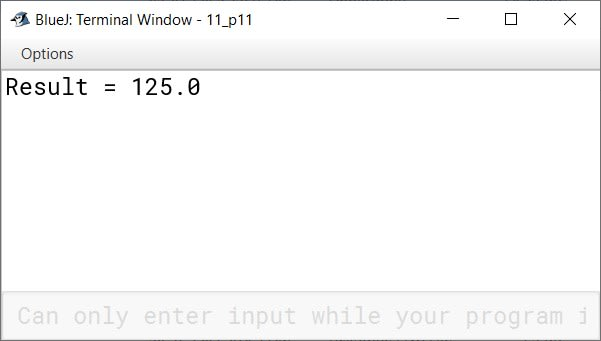#### Question 2

Write a program to calculate the gross salary of an employee when
Basic Salary = Rs.8600
DA = 20% of Salary
HRA = 10% of Salary
CTA = 12% of the Salary.
Gross Salary = (Salary + DA + HRA + CTA)
Display the basic and gross salary.

```public class KboatSalary
{
public static void main(String args[]) {
int sal = 8600;
double da = 20 / 100.0 * sal;
double hra = 10 / 100.0 * sal;
double cta = 12 / 100.0 * sal;
double gross = sal + da + hra + cta;
System.out.println("Gross Salary = " + gross);
}
}```
##### Output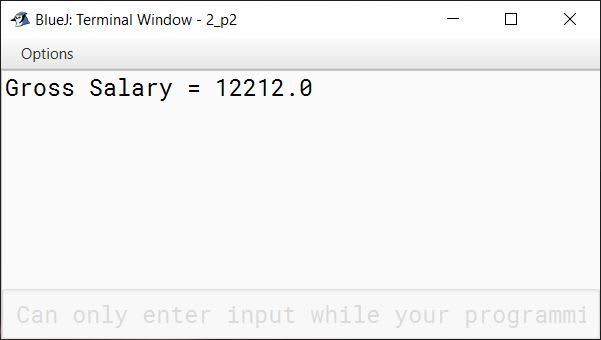#### Question 3

Write a program to accept Principal, Rate and Time. Calculate and display the interest accumulated for the first year, second year and the third year compound annually.

Sample Input:
Principal = ₹5,000, Rate = 10% per annum, Time = 3 years

Sample Output:
Interest for the first year: ₹500
Interest for the second year: ₹550
Interest for the third year: ₹605

```public class KboatInterest
{
public static void main(double p, double r, int t) {
double i1 = p * r * 1 / 100.0;
double amt = p + i1;
System.out.println("Interest for the first year: " + i1);

double i2 = amt * r * 1 / 100.0;
amt = amt + i2;
System.out.println("Interest for the second year: " + i2);

double i3 = amt * r * 1 / 100.0;
amt = amt + i3;
System.out.println("Interest for the third year: " + i3);
}
}```
##### Output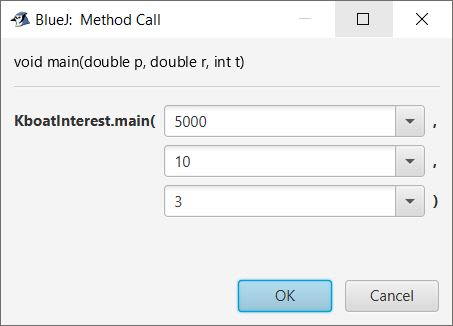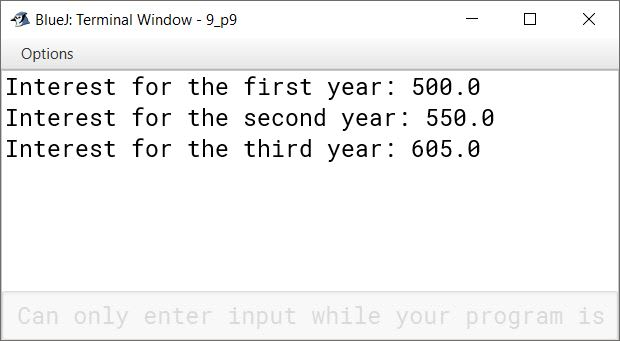#### Question 4

A shopkeeper announces two successive discounts 20% and 10% on purchasing of goods on the marked price. Write a program to input marked price. Calculate and display the selling price of the article.

```public class KboatSuccessiveDiscount
{
public static void main(double mp) {
double d1 = mp * 20 / 100.0;
double amt1 = mp - d1;

double d2 = amt1 * 10 / 100.0;
double amt2 = amt1 - d2;

System.out.print("Selling Price = " + amt2);
}
}```
##### Output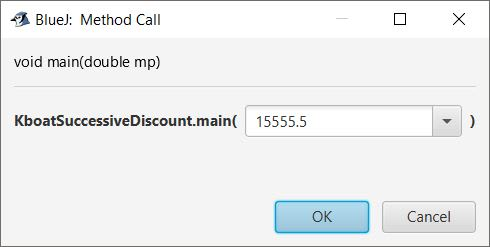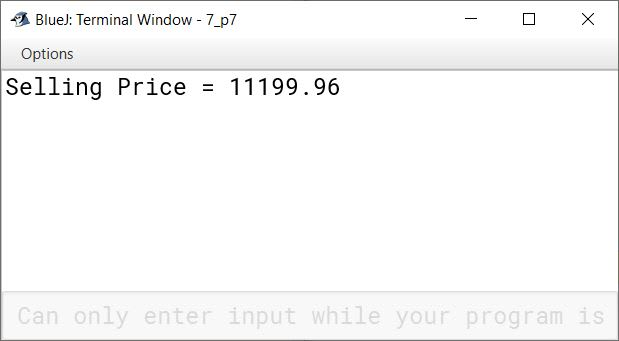#### Question 5

Write a program to input two unequal numbers. Display the numbers after swapping their values in the variables without using a third variable.
Sample Input: a=23, b= 56
Sample Output: a=56, b=23

```public class KboatNumberSwap
{
public static void main(int a, int b) {
a = a + b;
b = a - b;
a = a - b;

System.out.println("Numbers after Swap:");
System.out.println("a = " + a + "\t" + "b = " + b);
}
}```
##### Output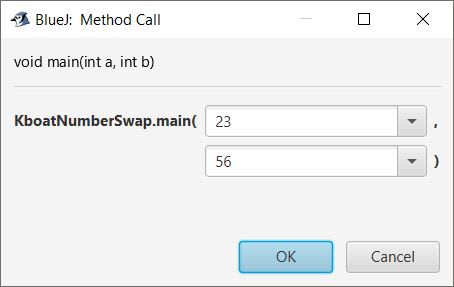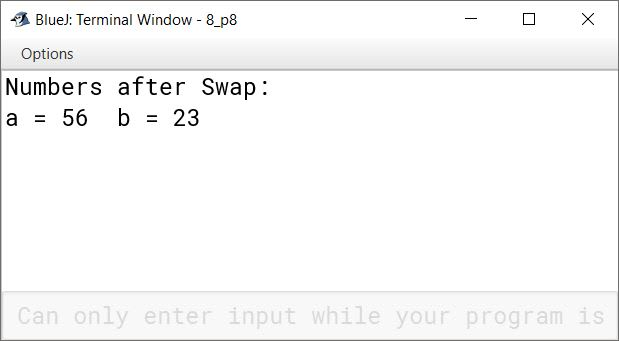#### Question 6

Write a program to input the temperature in Celsius and then convert it into Fahrenheit. If the temperature in Fahrenheit is more than 98.6°F then display "Fever", otherwise "Normal".

```public class KboatTemperature
{
public static void main(double cel) {

double far = 1.8 * cel + 32;

if (far > 98.6)
System.out.println("Fever");
else
System.out.println("Normal");
}
}```
##### Output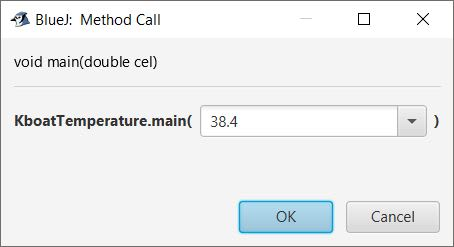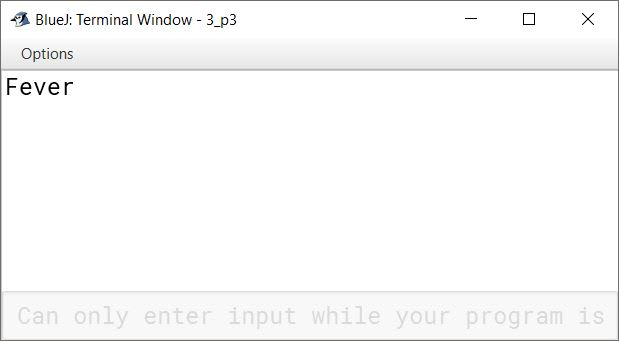#### Question 7

Write a program to accept the marks, obtained in five different subjects (English, Physics, Chemistry, Biology, Maths) by a student and find the average marks. If the average is 80 or more, then display he/she is eligible to get "Computer Science', otherwise "Biology".

```public class KboatMarks
{
public static void main(int eng, int phy, int chem,
int bio, int math)
{
double avg = (eng + phy + chem + bio + math) / 5.0;

if (avg >= 80) {
System.out.println("Eligible for Computer Science");
}
else {
System.out.println("Eligible for Biology");
}
}
}```
##### Output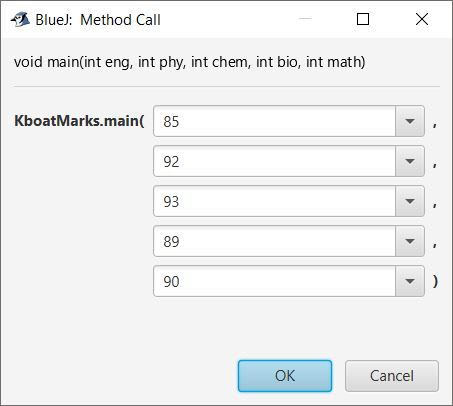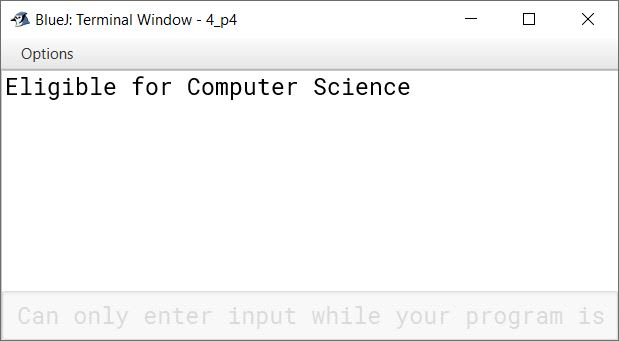#### Question 8

'Mega Market' has announced festival discounts on the purchase of items, based on the total cost of the items purchased:

Total costDiscount
Up to ₹2,0005%
₹2,001 to ₹5,00010%
₹5,001 to ₹10,00015%
Above ₹10,00020%

Write a program to input the total cost. Display the discount and amount to be paid after discount.

```public class KboatDiscount
{
public static void main(double c) {
int d = 0;

if (c <= 2000)
d = 5;
else if (c <= 5000)
d = 10;
else if (c <= 10000)
d = 15;
else
d = 20;

double disc = c * d / 100.0;
double amt = c - disc;

System.out.println("Discount: " + disc);
System.out.println("Amount to be paid: " + amt);
}
}```
##### Output